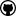org.dllearner.refinementoperators

## Class MathOperations

• ```public class MathOperations
extends Object```
Math operations related to refinement operators.
Author:
Jens Lehmann
• ### Constructor Summary

Constructors
Constructor and Description
`MathOperations()`
• ### Method Summary

All Methods
Modifier and Type Method and Description
`static boolean` `containsDoubleObjectSomeRestriction(org.semanticweb.owlapi.model.OWLClassExpression d)`
Returns true if the same property is used twice in an object some restriction, e.g.
`static List<List<Integer>>` `getCombos(int number)`
Given `number`, the functions returns all combinations of natural numbers plus the number count (which can be thought of as the number of interconnecting symbols between those numbers) adds up to `number`.
`static List<List<Integer>>` ```getCombos(int length, int maxValue)```
Methods for computing combinations with the additional restriction that `maxValue` is the highest natural number, which can occur.
`static SortedSet<org.semanticweb.owlapi.model.OWLObjectUnionOf>` ```incCrossProduct(Set<org.semanticweb.owlapi.model.OWLObjectUnionOf> baseSet, Set<org.semanticweb.owlapi.model.OWLClassExpression> newSet)```
Implements a cross product in the sense that each union in the base set is extended by each class expression in the new set.
• ### Methods inherited from class java.lang.Object

`equals, getClass, hashCode, notify, notifyAll, toString, wait, wait, wait`
• ### Constructor Detail

• #### MathOperations

`public MathOperations()`
• ### Method Detail

• #### getCombos

`public static List<List<Integer>> getCombos(int number)`
Given `number`, the functions returns all combinations of natural numbers plus the number count (which can be thought of as the number of interconnecting symbols between those numbers) adds up to `number`. It uses an efficient algorithm to achieve this, which can handle number=50 in less than a second and number=30 in about 10 milliseconds on an average PC. For illustrating the function, the return values of the first numbers are given: number = 1: [] number = 2: [] number = 3: [, [1, 1]] number = 4: [, [2, 1]] number = 5: [, [3, 1], [2, 2], [1, 1, 1]] number = 6: [, [4, 1], [3, 2], [2, 1, 1]] number = 7: [, [5, 1], [4, 2], [3, 3], [3, 1, 1], [2, 2, 1], [1, 1, 1, 1]]
Parameters:
`number` - A natural number.
Returns:
A two dimensional list constructed as described above.
• #### getCombos

```public static List<List<Integer>> getCombos(int length,
int maxValue)```
Methods for computing combinations with the additional restriction that `maxValue` is the highest natural number, which can occur.
Parameters:
`length` - Length of construct.
`maxValue` - Maximum value which can occur in sum.
Returns:
A two dimensional list constructed in `getCombos(int)`.
`getCombos(int)`
• #### incCrossProduct

```public static SortedSet<org.semanticweb.owlapi.model.OWLObjectUnionOf> incCrossProduct(Set<org.semanticweb.owlapi.model.OWLObjectUnionOf> baseSet,
Set<org.semanticweb.owlapi.model.OWLClassExpression> newSet)```
Implements a cross product in the sense that each union in the base set is extended by each class expression in the new set. Example: baseSet = {A1 OR A2, A1 or A3} newSet = {A1, EXISTS r.A3} Returns: {A1 OR A2 OR A1, A1 OR A2 OR EXISTS r.A3, A1 OR A3 OR A1, A1 OR A3 OR EXISTS r.A3} If the base set is empty, then the return value are union class descriptions for each value in newSet (a union with only one concept).
Parameters:
`baseSet` - A set of union class descriptions.
`newSet` - The descriptions to add to each union class descriptions.
Returns:
The "cross product" of baseSet and newSet.
• #### containsDoubleObjectSomeRestriction

`public static boolean containsDoubleObjectSomeRestriction(org.semanticweb.owlapi.model.OWLClassExpression d)`
Returns true if the same property is used twice in an object some restriction, e.g. (EXISTS r.A1 AND A2 AND EXISTS r.A3) returns true, while (A1 OR A2) and (EXISTS r.A1 AND A2 AND EXISTS s.A3) return false. Note that the method does not work recursively, e.g. it return false for EXISTS r.(EXISTS r.A1 AND A2 AND EXISTS r.A3).
Parameters:
`d` - OWLClassExpression to test.
Returns:
See description.DL-Learner is licenced under the terms of the GNU General Public License.